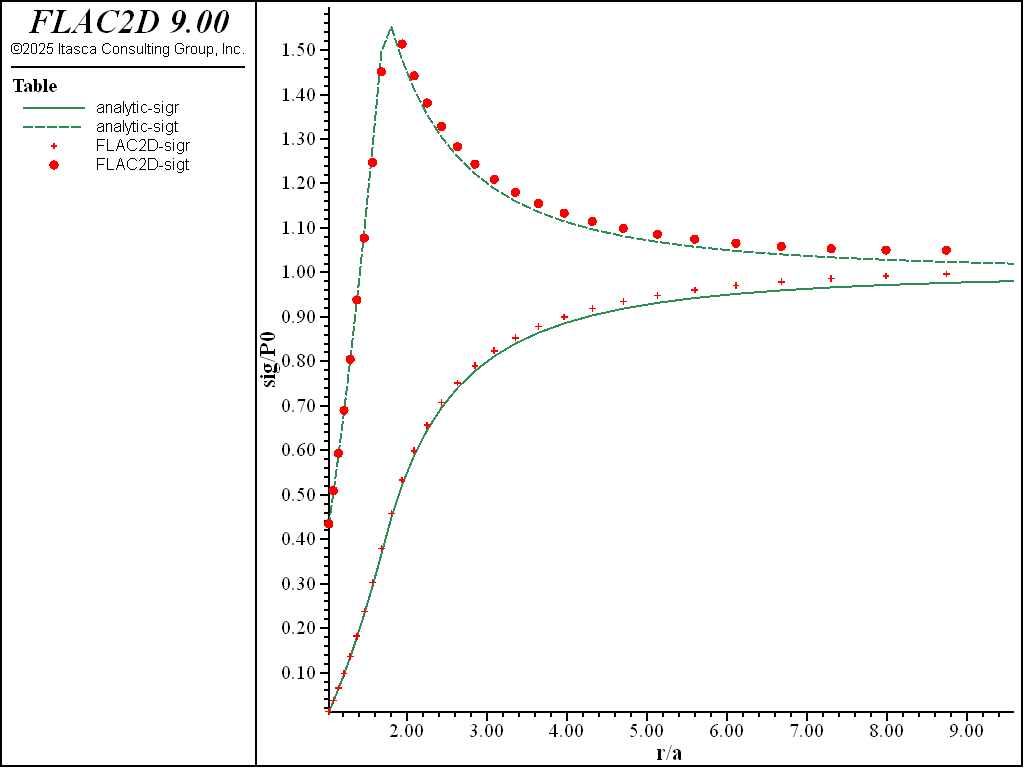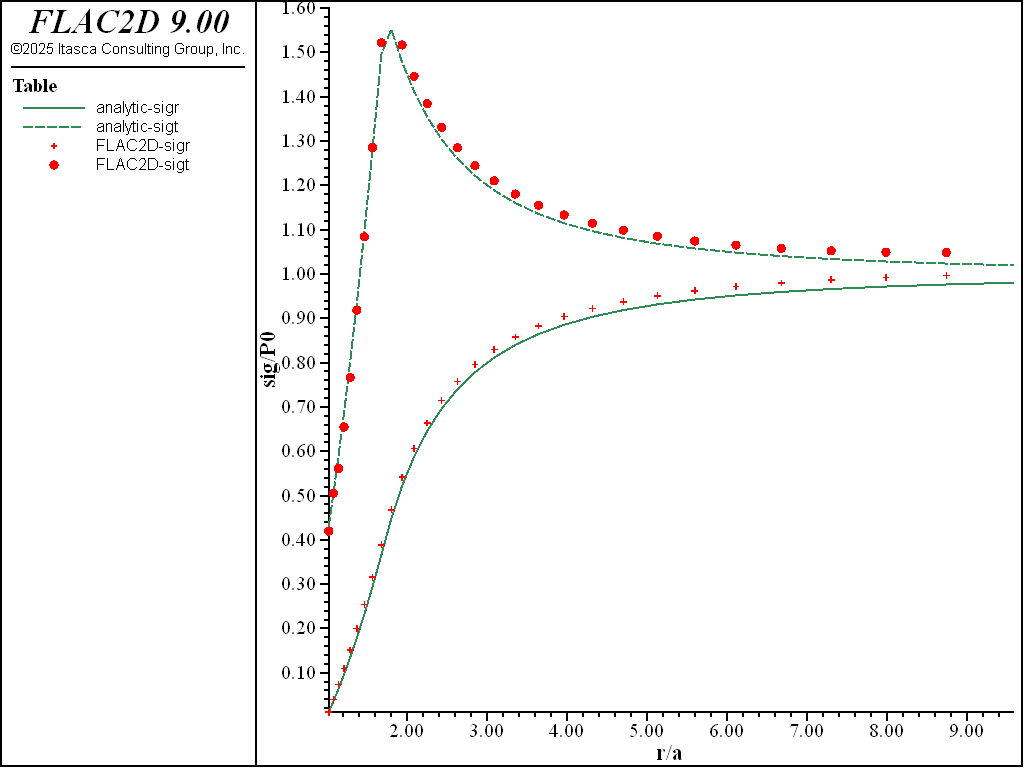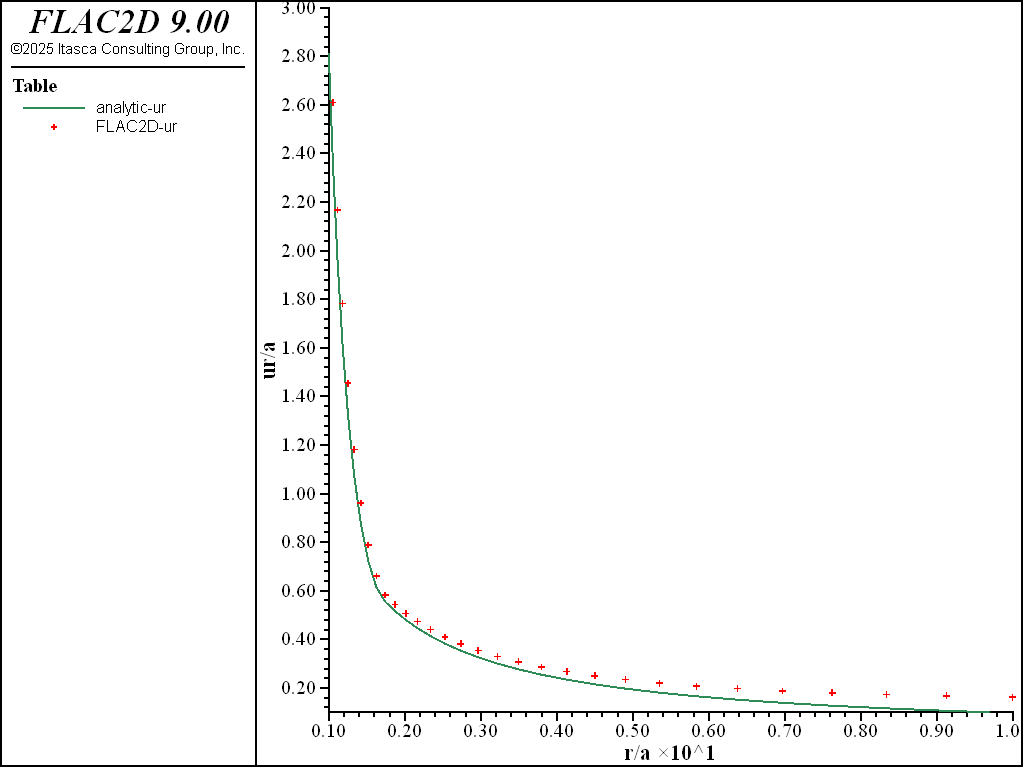Examples • Verification Problems

# Cylindrical Hole in an Infinite Mohr-Coulomb Material (FLAC2D)

Problem Statement

Note

To view this project in FLAC2D, use the menu command Help ► Examples…. Choose “VerificationProblems/ CylinderInMohrCoulomb” and select “CylinderInMohrCoulomb.prj” to load. A representative sample of the data files used for structured qadrilateral grid and associated flow is shown at the end of this example. The remaining data files can be found in the project.

Stresses and displacements are determined numerically for the case of a cylindrical hole in an infinite elasto-plastic material subjected to in-situ stresses. The material is assumed to be linearly elastic, perfectly plastic, with a failure surface defined by the Mohr-Coulomb criterion, and both associated (dilatancy = friction angle) and nonassociated (dilatancy = 0) flow rules are used. The results of the simulation are compared with an analytic solution.

This problem tests the Mohr-Coulomb plasticity model under plane-strain conditions and it is solved numerically in FLAC2D.

The Mohr-Coulomb material is assigned several properties:

 shear modulus ($$G$$) 2.8 GPa bulk modulus ($$K$$) 3.9 GPa cohesion ($$c$$) 3.45 MPa friction angle ($$\phi$$) 30° dilation angle ($$\psi$$) 0° and 30°

The isotropic in-situ stress has a magnitude of -30 MPa, and the pressure inside the hole may be neglected. (As a convention, compressive stresses are negative.)

Closed-Form Solution

The analytic solution for this problem may be found in Salençon (1969). The yield zone radius, $$R_0$$, may be expressed, in general terms, as

${{R_0}\over{a}}\ =\ \left [{ {2 \over {K_p+1}}\ \ { {1+ {{q}\over{P_0}}\ k_p} \over {{{P_i}\over{P_0}} + {q \over{P_0}}\ k_p} } }\right ]^{k_p}$

in which $$a$$ is the hole radius, $$P_0$$ is the absolute value of the in-situ isotropic stress, $$P_i$$ is the pressure inside the hole (0 Pa, in this case), and

$\begin{split}\begin{split} K_p\ &=\ {{1+\sin \phi} \over {1-\sin \phi}} \\ k_p\ &=\ {1 \over {K_p-1}} \\ q\ &=\ 2 \cdot c \cdot \sqrt{K_p} \end{split}\end{split}$

The radial stress at the elastic/plastic interface may be written as

${{\sigma_{re}}\over{P_0}}\ =\ -\ {1\over{K_p+1}}\ \left ({2 - {q\over{P_o}} }\right )$

The stresses in the plastic zone have the form

$\begin{split}\begin{split} {{\sigma_r}\over{P_0}}\ &=\ {q\over{P_0}}\ k_p\ -\ \left ({{{P_i}\over{P_0}}\ +\ {q\over{P_0}}\ k_p }\right ) \left ({{r\over a} }\right ) ^{K_p-1} \\ {{\sigma_\theta}\over{P_0}}\ &=\ {q \over {P_0}}\ k_p\ -\ K_p\ \left ({ {{P_i}\over{P_0}}\ +\ {q\over{P_0}}\ k_p }\right ) \left ({ {r\over a} }\right ) ^{K_p-1} \end{split}\end{split}$

in which $$r$$ is the distance from the hole axis.

The stresses in the elastic zone are

${{\sigma_r}\over{P_0}}\ =\ -1\ +\ \left ({ 1\ -\ {{\sigma_{re}}\over{P_0}} }\right ) \left ({ {{R_0}\over a}\ {a\over r} }\right ) ^2$
${{\sigma_\theta}\over{P_0}}\ =\ -{{\sigma_r}\over{P_0}}\ -\ 2$

The displacements in the elastic region are given as

${{u_r}\over a}\ =\ -{{P_0}\over{2G}} \left ({ 1\ +\ {{\sigma_{re}}\over{P_0}} }\right) \left ({ {{R_o}\over a} }\right ) ^2\ \left ({{a\over r} }\right )$

and, in the plastic region, as

${{u_r}\over a}\ =\ -{{P_0}\over{2G}}\ {r\over a}\ \chi \left ({ {r\over a} }\right )$

in which

$\begin{split}\chi \left({ {r\over a} }\right ) & =\ \bigl (2\nu - 1)\ (1 + {q \over {P_0}}\ k_p \bigr ) \\ & +\ {{(1-\nu)(K^2_p - 1)} \over {K_p + K_{ps}}}\ \left ({ {{P_i}\over{P_0}}\ +\ {q \over{P_0}}\ k_p }\right )\ \left ({ {{R_0}\over a} }\right )^{K_p+K_{ps}}\ \left ({ {r\over {R_0}} }\right )^{-K_{ps}-1} \\ & +\ \left [{ (1-\nu)\ {{K_p\ K_{ps} + 1}\over{K_p + K_{ps}}}\ -\ \nu }\right ] \ \left ({ {{P_i}\over{P_0}}\ +\ {q \over{P_0}}\ k_p }\right )\ \left ({ {r \over a} }\right )^{K_p -1}\end{split}$

and

$K_{ps}\ =\ {{1+\sin \psi} \over {1-\sin \psi}}$

In these equations, $$\nu$$ is Poisson’s ratio, $$\psi$$ is the dilation angle and $$G$$ is the shear modulus.

FLAC2D Model

For modeling purposes, the problem is defined by the domain presented in Figure 1, where advantage has been taken of the quarter-symmetry geometry. The boundary conditions applied to this domain are also shown in Figure 1. The domain is discretized into 900 zones organized in a radial pattern (for the case of structured quadrilateral grid).

In the numerical simulation, the initial stress state (corresponding to $$P_0$$ = 30 MPa) is applied throughout the domain.Figure 1: Domain, grid, and boundary conditions for FLAC2D simulation—quarter symmetry.

Results and Discussion

Figure 2 through Figure 5 show comparisons between FLAC2D results and the analytic solution along a radial line. Normalized stresses, $$-{\sigma}_r/P_0$$ and $$-{\sigma}_{\theta}/P_0$$, are plotted versus normalized radius, $$r/a$$, in Figure 2 and Figure 3, while normalized displacements, $$-u_r/a$$, are represented versus $$r/a$$ in Figure 4 and Figure 5.Figure 2: Stress solution comparison—associated (analytical values = lines; numerical values = crosses/circles).Figure 3: Stress solution comparison—nonassociated (analytical values = lines; numerical values = crosses/circles).Figure 4: Radial displacement solution comparison—associated (analytical values = lines; numerical values = crosses).Figure 5: Radial displacement solution comparison—nonassociated (analytical values = lines; numerical values = crosses).

The average relative error on the stresses and displacements is less than 3% throughout the grid. (Note that the error could be reduced by more appropriate handling of the far field conditions using, for example, the Lamé solution for a thick ring.)

Displacement contours and displacement vectors for the associated case are presented in Figure 6, as an illustration.

The file “associated.dat” used to generate the associated case is shown below and “non-associated.dat” is different only in the dilatation value assigned and can be found in the project. (The dilatation angle is 30° in the associated case.)

The files “associated-nmd.dat”, “non-associated-nmd.dat” (triangular grid) and “associated-nmd-mix.dat”, “non-associated-nmd-mix.dat” (mixed grid) generate the same two cases, but using FLAC2D’s nodal mixed discretization (NMD) feature and unstructured grids with triangular zones only or mixed quadrilateral-trianglar zones, respectively (grids are imported from files “nmd_tri.f2grid” and “nmd_mix.f2grid”). The NMD calculations are automatically executed for all triangular zones if NMD option is not explicitly specified (however, it can be turned on or off via zone nodal-mixed-discretization command). For illuistration purpoces, only the case of the triangular grid is shown below.

The file “sale.dat” compares the numerical solutions to the analytical solution using FISH functions:

1. nastr calculates (i) numerical and corresponding analytical values of $$-{\sigma}_r/P_0$$ and $$-{\sigma}_{\theta}/P_0$$ at the centroid of zones closest to the $$x$$-axis; and (ii) average relative error of $$-{\sigma}_r/P_0$$ and $$-{\sigma}_{\theta}/P_0$$ throughout the grid.

2. nadis calculates (i) numerical and analytical values of $$u_r/a$$ at gridpoints located on the $$x$$-axis; and (ii) the average relative error of the displacement $$u_r/a$$ throughout the grid.

The resulting errors are within 3% of the analytical solution for stresses and displacements for both associated and nonassociated flow rules for triangular grid. Figure 7 through Figure 10 show the NMD results and correspond directly with Figure 2 through Figure 6 (results with an all-quad grid). As can be seen, the results are very similar.

Reference

Salençon, J. “Contraction Quasi-Statique D’une Cavite a Symetrie Spherique Ou Cylindrique Dans Un Milieu Elastoplastique,” Annales Des Ponts Et Chaussees, 4, 231-236 (1969).

Data File

associated.dat

;---------------------------------------------------------------------
;            numerical solution for a long tunnel in pre-stressed
;             Mohr Coulomb material (salencon problem)
;             associated and non-associated plastic flow
;---------------------------------------------------------------------
model new
model large-strain off
; Create zones
zone create sector-quad size 1 30 30 rat 1 1 1.1 point 1 10 0 point 2 0 10 dim 1 1
; Assign constitutive model and properties
zone cmodel assign mohr-coulomb
zone property bulk 3.9e9 shear 2.8e9 cohesion 3.45e6
zone property friction 30. dilation 30. tension 1.e10 ; associated flow
; Initialize stress
zone initialize stress xx -30e6 yy -30e6 zz -30e6
; Apply boundary conditions
zone face apply velocity-normal 0.0 range union position-x 0 position-y 0
zone face apply stress-normal -30e6 range union position-x 10 position-y 10
; Take some histories
zone history displacement-x position 1 0
zone history velocity-x position 1 0
zone history unbalanced-force-y position 1 0
history interval 20
; Solve
model solve ratio-local 1e-4
; Save the model
model save 'associated'